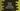# How to create a dictionary from two lists in python## Create a dictionary from two lists in python :

In this python programming tutorial, we will learn how to create a dictionary from two different user input lists. Our program will ask the user to enter the values for both lists and then it will create one dictionary by taking the values. Values of the first list will be the key to the dictionary and corresponding values of the second list will be the value of the dictionary.

## Python dictionary :

Dictionaries are used to store key-value pairs in python. Dictionaries are unordered collections. We can access any items in a dictionary by referring its key name. Using a key, we can also change the value in a dictionary.

Dictionary is defined by enclosing all key-value pairs in curly braces({}). A colon (:) is used to separate a key from its value.

### Python list :

Python list is created by enclosing all items inside a square bracket ([]). Each item are separated by a comma. Lists are similar to dictionaries. We can access any element using its index, we can modify or read an element.

Both dictionary and lists are mutable and dynamic.

In this tutorial, you will learn how to populate lists by taking user provided values, how to print a list to the user, how to create a dictionary using two lists and how to print that dictionary.

### Python program :

``````#1
first_list = []
second_list = []
#2
first_list_size = int(input("Enter total elements for the first list : "))
second_list_size = int(input("Enter total elements for the second list : "))
#3
for i in range(first_list_size):
first_list.append(input("Enter value for the first list : "))
#4
for i in range(second_list_size):
second_list.append(input("Enter value for the second list : "))
#5
#6
combined_dict = dict(zip(first_list,second_list))
#7
print("Final dictionary : ",combined_dict)``````

### Explanation :

The commented numbers in the above program denote the step number below :

1. Create two empty lists firstlist_ and secondlist_ to store the values for the keys and values of the dictionary. We will read all list values from the user.
2. Ask the user to enter the size of both the lists. Read and store them in firstlistsize and secondlistsize variables. Note that the size should be the same for both lists. Because the first list will hold the keys and the second list will hold the values for the dictionary. The count of keys and values should be the same.
3. Run one for loop. Take the values for the first list from the user and store them in firstlist_ list one by one.
4. Similarly, using one for loop, read and store the values for the second list in secondlist_ variable.
5. Print out both lists to the user.
6. Using zip(), create one list of pairs from the lists. Then using dict(), convert that list of pairs to a dictionary.
7. Print out the created dictionary.

### Conclusion :

Zipping is the easiest way to convert two lists to a dictionary. The first example we have shown above is a simple one, but the second and third examples are a little bit different. Both have different numbers of items in the lists. You can see that the final dictionary doesn’t contain all items.zip() ignores the items of a list if it doesn’t have anything corresponding in the other list. Go through the example and drop a comment below if you have any queries.